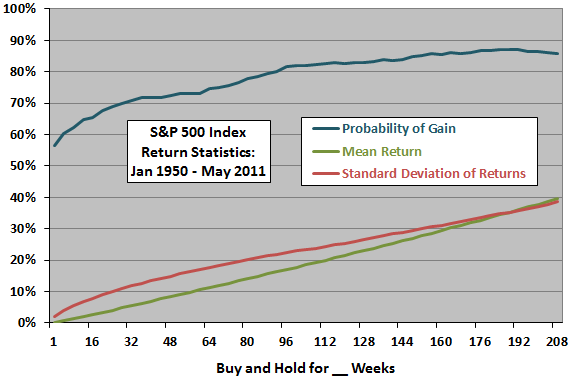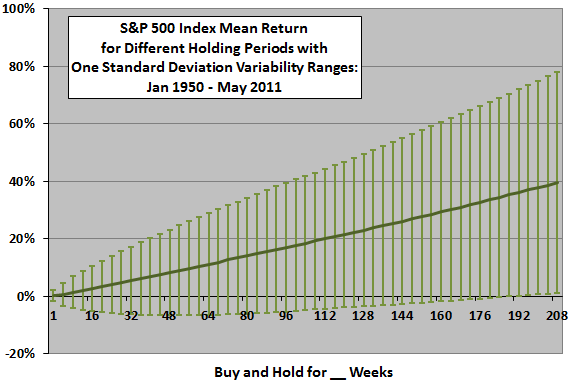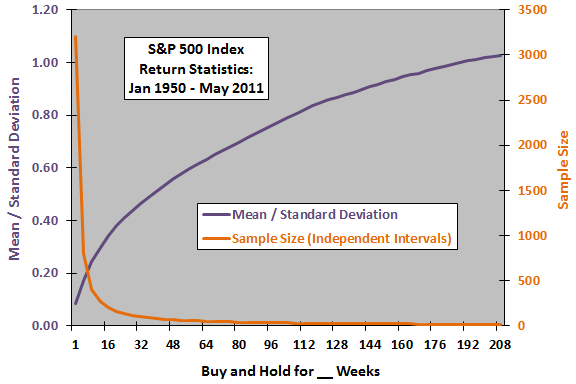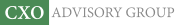Objective research to aid investing decisions

#### Value Investing Strategy (Strategy Overview)

Allocations for December 2021 (Final)
Cash TLT LQD SPY

#### Momentum Investing Strategy (Strategy Overview)

Allocations for December 2021 (Final)
1st ETF 2nd ETF 3rd ETF

# Notes on Variability of Stock Market Returns

| | Posted in: Big Ideas, Equity Premium, Volatility Effects

How should the variability of stock market returns shape the outlooks of short-term traders and long-term investors? How strong is the tailwind of the general drift upward in stock prices? How powerful is the turbulence of variability? Does the tailwind ever overpower the turbulence? Using weekly closes for the S&P 500 Index during for January 1950 through May 2011 (3,204 weeks or about 61 years), we find that:

The following chart presents three statistics for random S&P 500 Index entry points and holding periods from one week to 208 weeks (four years) over the entire sample period. The chart shows that:

(1) The likelihood of a gain in the index ranges from 56.5% for a typical one-week holding period to around 87% for typical holding periods approaching four years. (The probability of gain for shorter and shorter holding periods less than one week trends down toward 50%.)

(2) The mean return ranges from less than 0.2% for a one-week holding period to nearly 40% for a four-year (208-week) holding period.

(3) The standard deviation of returns is mostly higher, but increases more slowly, than the mean return. The mean crosses above the standard deviation as the holding period approaches four years. Said differently, turbulence swamps the tailwind for short holding periods, but not for long holding periods.The next chart provides another perspective on the relationship between mean return and standard deviation of returns for random S&P 500 Index entry points and varying holding periods over the entire sample period, by depicting standard deviation as a variability range around the mean. The chart shows that:

(1) For short holding periods, a variability range of one standard deviation encompasses a significant proportion of losses.

(2) For long holding periods, a variability range of one standard deviation encompasses a very small proportion of losses (no losses for holding periods 188 weeks and longer). For higher confidence levels (variability ranges larger than one standard deviation), however, no-loss holding periods would be longer.

If returns are normally distributed, these error ranges represent 68% confidence intervals (returns for 68% of a large sample of randomly selected entry points for each holding period would fall within the error ranges). However, these returns are likely not normally distributed (probably more fat-tailed and skewed), so a range of one standard deviation equates to a confidence somewhat different from 68%. For example, assuming a normal distribution and a 188-week holding period, the estimated probability of gain is about 68% + 32%/2 = 84%. However, the actual probability of gain is 87.2%.The final chart displays two additional statistics for random S&P 500 Index entry points and varying holding periods over the entire sample period. The chart shows that:

(1) The ratio of the mean return to the standard deviation of returns (return per unit of risk, something like the Sharpe ratio), increases steadily from below 0.1 (turbulence >> tailwind) for a one-week holding period to over 1.0 (turbulence ~ tailwind) for a four-year holding period.

(2) However, the number of independent observations within the sample falls quickly (initially much faster than the ratio of mean to standard deviation rises) as the holding period lengthens. For a four-year holding period, the number of independent observations is only 14. The decline in sample size tends to undermine the apparent comfort of long holding periods.In summary, evidence from simple analysis of U.S. stock market returns shows that shrinkage of effective sample size undermines confidence in the reward-per-unit-of-risk benefit of a lengthening investment horizon.

Also, stock market direction forecasters could have achieved high accuracy rates by making only long-term, bullish predictions. (The Guru Grades approach takes this bullish bias into account by detrending.)

Cautions regarding findings include:

• The index used excludes the varying effect of dividends.
• Factors such as legal/regulatory regime, demographics and raw resource availability arguably drive the long-term upward drift in U.S. stock prices. Sample size in the context of such drivers may be extremely small.
• Conventional interpretations of the mean and standard deviation assume a normal (Gaussian) distribution of returns. To the extent that the actual return distribution is wild rather than tamely normal, these interpretations lose predictive power.